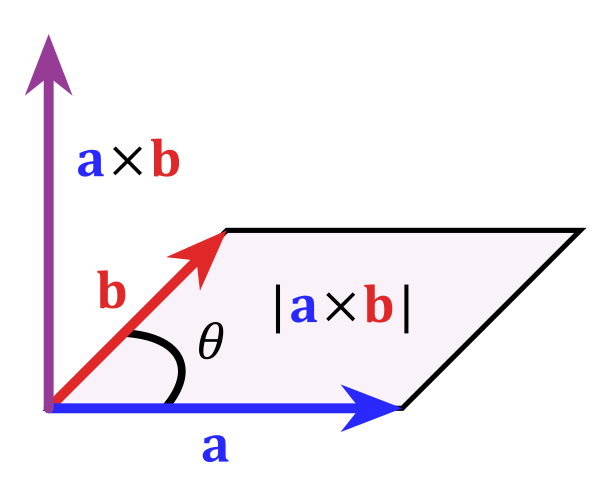Math Vector algebra Cross product/Vector product

# Cross product

In addition to the scalar product, there is the cross product (or vector product) of two vectors. As a result you get a vector that is perpendicular to the other vectors.

Mathematically, the cross product can be calculated as follows:

$\vec{a}\times\vec{b}$ $=\begin{pmatrix}a_1\\a_2\\a_3\end{pmatrix}\times\begin{pmatrix}b_1\\b_2\\b_3\end{pmatrix}$ $=\begin{pmatrix}a_2\cdot b_3-a_3\cdot b_2\\a_3\cdot b_1-a_1\cdot b_3\\a_1\cdot b_2- a_2\cdot b_1\end{pmatrix}$
i

### Info

The magnitude of the vector $\vec{c}=\vec{a}\times\vec{b}$ equals the surface area of the parallelogram formed by $\vec{a}$ and $\vec{b}$.

$A=|\vec{c}|$ $=|\vec{a}\times\vec{b}|$i

### Hint

The cross product is often a quick alternative to calculate a normal vector for a normal equation.

However, this formula is hard to memorize so sometimes it is not taught in school.

### Example

$\begin{pmatrix}a_1\\a_2\\a_3\end{pmatrix}\times\begin{pmatrix}b_1\\b_2\\b_3\end{pmatrix}$ $=\begin{pmatrix}a_2\cdot b_3-a_3\cdot b_2\\a_3\cdot b_1-a_1\cdot b_3\\a_1\cdot b_2- a_2\cdot b_1\end{pmatrix}$

$\begin{pmatrix} 1 \\ 1 \\ 0 \end{pmatrix} \times\begin{pmatrix} 1 \\ 5 \\ 2 \end{pmatrix}$ $=\begin{pmatrix} 1\cdot2 - 0\cdot5 \\ 0\cdot1 - 1\cdot2 \\ 1\cdot5 - 1\cdot1 \end{pmatrix}$ $=\begin{pmatrix} 2 \\ -2 \\ 4 \end{pmatrix}$